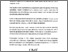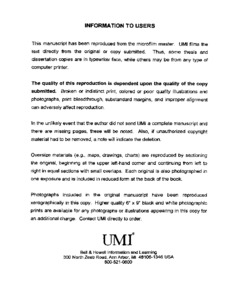Title:

# The Fourier-Stieltjes transform and absolutely continuous invariant measures

Gómez-Nesterkín, Ruslán (1999) The Fourier-Stieltjes transform and absolutely continuous invariant measures. Masters thesis, Concordia University.Preview Text (application/pdf) MQ39074.pdf 1MB

## Abstract

We present some results on the existence of absolutely continuous invariant measures (acim's) using the Fourier-Stieltjes transform. We consider the sequence of Perron Frobenius operators $$\{f,P\sb{\tau}f,P\sbsp{\tau}{2}f,P\sbsp{\tau}{3}f,\... ,P\sbsp{\tau}{n}f,\...\},$$induced by the nonsingular transformation $\tau:I\to I,$ with $f \in {\cal L}\sp1$, and the associated sequence of Fourier-Stieltjes transforms$$\{ {\cal F}(F\sb0), {\cal F}(F\sb1), {\cal F}(F\sb2),\...,{\cal F}(F\sb{n}),\...\},$$where $F\sb{n}(x) = f\sbsp{-\infty}{x}\ P\sbsp{\tau}{n}f(u)du$ and ${\cal F}(F\sb{n})(t) = f\sbsp{-\infty}{\infty}\ e\sp{itx}dF\sb{n}(x).$ The main result is: if $\tau$ is piecewise monotonic expanding and ${1\over{{d\over dx}\tau(\cdot)}}$ is a function of bounded variations, then $\tau$ has an acim. Although this is a known result, the method of proof is new and may allow generalizations needed. Finally, we introduce criteria on the Fourier Stieltjes Transforms needed to ensure the existence of acim's.

Divisions: Concordia University > Faculty of Arts and Science > Mathematics and Statistics Thesis (Masters) Gómez-Nesterkín, Ruslán v, 49 leaves ; 29 cm. Concordia University M.Sc. Mathematics 1999 Gora, Pawel QA 403.5 G66 1999 719 Concordia University Library 27 Aug 2009 17:13 13 Jul 2020 19:47 https://concordiauniversity.on.worldcat....
All items in Spectrum are protected by copyright, with all rights reserved. The use of items is governed by Spectrum's terms of access.

Repository Staff Only: item control pageResearch related to the current document (at the CORE website)
Back to top